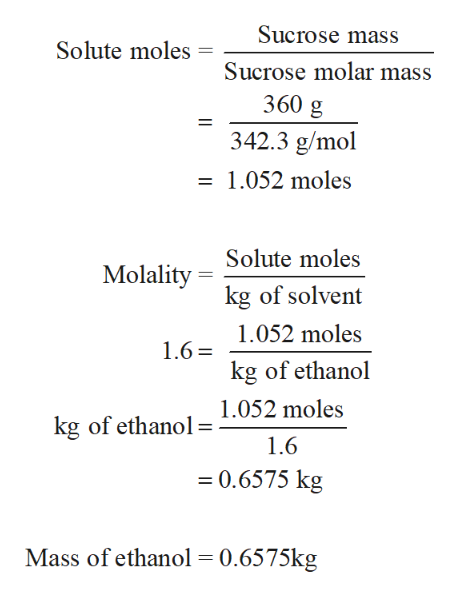# What mass of ethanol if 360 g if sucrose are dissolved in ethanol to make a 1.6 molal solution? If the density of ethanol is 0.89 g/mL, determine the volume of ethanol used.

Question
12 views

What mass of ethanol if 360 g if sucrose are dissolved in ethanol to make a 1.6 molal solution? If the density of ethanol is 0.89 g/mL, determine the volume of ethanol used.

check_circle

Step 1

First, using given molality and mass of sucro...help_outlineImage TranscriptioncloseSucrose mass Solute moles Sucrose molar mass 360 g 342.3 g/mol = 1.052 moles Solute moles Molality kg of solvent 1.052 moles 1.6 = kg of ethanol 1.052 moles kg of ethanol= 1.6 = 0.6575 kg Mass of ethanol = 0.6575kg fullscreen

### Want to see the full answer?

See Solution

#### Want to see this answer and more?

Solutions are written by subject experts who are available 24/7. Questions are typically answered within 1 hour.*

See Solution
*Response times may vary by subject and question.
Tagged in

### General Chemistry